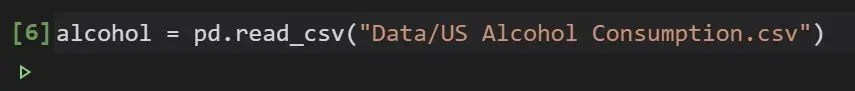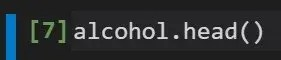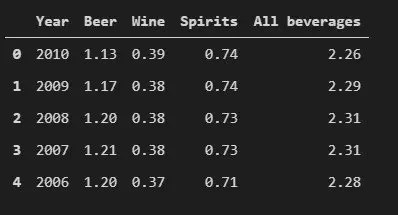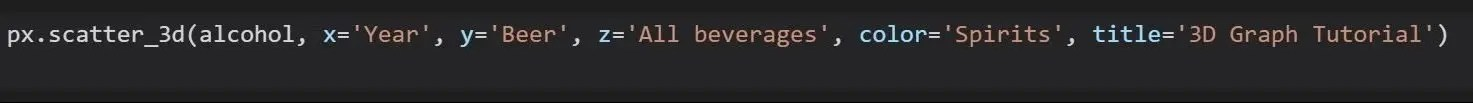# How to Create a 3D Graph in Python using Plotly Express

### How to Create a 3D Graph in Python using Plotly Express

This tutorial is going to show you how to create a 3d graph in python using the plotly express library. Let’s dive in.

### Step 2: Import plotly express and pandas.

After installing the plotly express library, you import it into your notebook and also import pandas. Pandas will be used to load and read the dataset while plotly express is used to create the 3d graph.### Step 3: Load the dataset that you want to plot

You use pandas read csv function to load the csv file and read it. Then store this loaded dataset to a variable (alcohol in this code below).### Step 4: Verify that the data is loaded correctly.

This is the code to view the first 5 rows of the datasetThis is what the first 5 sections of the dataset looks like.### Step 5: Plot the dataset that you want using the following lines of code.• alcohol(dataframe) is the first parameter you put in for a plotly express graph. The dataset you are graphing is always the initial parameter.
• X = the data that goes on the X-axis
• Y = the data that goes on the Y-axis
• Z = the data that goes on the Z-axis
• Color = Which column you want to use to define the color, color has to be the name of a dataset.
• Title = title for the graph

You can add other parameters to your 3d graph like color, size,  opacity, size_max, symbol, e.t.c. to access other features.

This is an example of what a scatter 3d graph might look like when you are done.